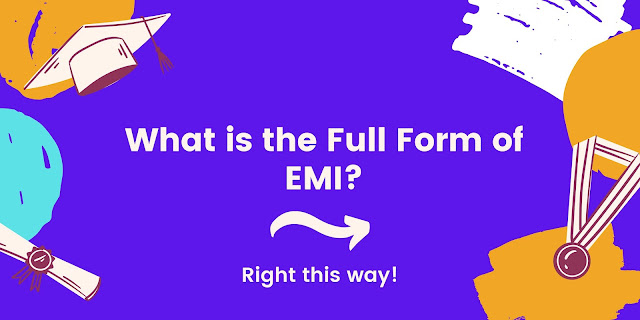What is the full form of EMI, EMI Calculation?In this article, we will learn about the term EMI Full Form. On Google, search engine users search about What is the full form of EMI, EMI long-form, the full form of EMI, EMI full name, What is EMI full form.

From all these keywords user's intent is to know EMI's full form. The user wants to know what EMI is and how it is calculated and work in financial terms. Here you will gain some knowledge about EMI for information purposes.

Must Read: Full Form of HP.

What is the full form of EMI?

EMI long-form is Equated Monthly Installment. Most of us have heard about EMI. EMI is a term is coined in the financial field like in the Banking sector. Whenever you have visited malls and stores in any online stores, you have come across this EMI term. It is used when a person borrows some money from another person on fixed interest on a particular fixed date of every month.

What is EMI

EMI is Equated Monthly Installment. In simple terms, a Moneylender gets a sum of a fixed amount from a borrower with fixed interest on a particular date every month. EMI consist of the Moneylender, borrower, principal amount, fixed interest rate, and a particular ensure.

How is EMI Calculated?

EMI calculation is dependent on three factors:

1) Interest Rate: Amount of interest charged by the moneylender, for example. Bank.

2) Loan Amount ( principal loan): The amount borrowed.

3) Tenure of the Loan: Tenure is the time period given the borrower to repay the entire loan including the interest.

To calculate an EMI you can use these two:

1) flat rate method:

2) The reducing balance method

Use this formula to calculate  EMI reducing-balance method:

EMI = P * [( r * (1 + r)^n)) / ((1 + r)^n - 1)]

where:

P = Principal amount borrowed

r = Periodic monthly interest rate

n = Total number of monthly payments

TIPS: The EMI reducing-balance method often works out to be more cost-friendly to borrowers. The flat-rate method results in a higher effective interest rate.

1) Flexibility: EMI is a flexible financial method to borrow money. The different bank offers different interest rate with the different time period.

2) Power to Buy: It gives you to buy anything in installments.

FAQs Equated Monthly Installment (EMI):

1) What is EMI Full Form?

EMI stands for Equated Monthly Installment. In the financial world, EMI means an amount to be paid atw34 a particular time with a particular interest.

2) How to calculate EMI?

EMI can be calculated with two methods, first with the flat-rate method and second with the reducing-balance method.

3) EMI calculation is depended on which factors.

EMI calculation is dependent on three factors, Interest Rate, Loan Amount, Time Period.

4) Is EMI Good?

EMI is neither Good nor Bad. It depends on the condition of the borrower.

At the End of the post of EMI Full Form.

EMI full form is Equated Monthly Installment.

HPLC

SAARC

IPCC

EMI

DP

Previous
Next Post »